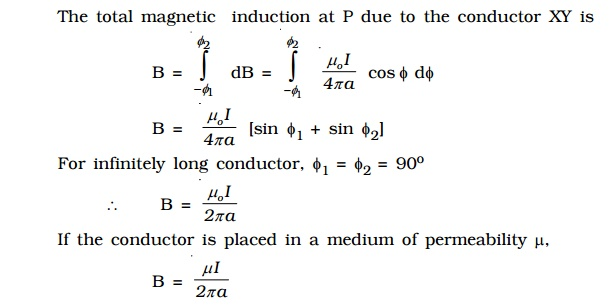Home | | Physics | Biot - Savart Law

# Biot - Savart LawBiot and Savart conducted many experiments to determine the factors on which the magnetic field due to current in a conductor depends. The results of the experiments are summarized as Biot-Savart law.

## Biot - Savart Law

Biot and Savart conducted many experiments to determine the factors on which the magnetic field due to current in a conductor depends.The results of the experiments are summarized as Biot-Savart law.

Let us consider a conductor XY carrying a current I (Fig 3.10). AB = dl is a small element of the conductor. P is a point at a distance r from the mid point O of AB. According to Biot and Savart, the magnetic induction dB at P due to the element of length dl is

1.     directly proportional to the current (I)

2.     directly proportional to the length of the element (dl )

3.     directly proportional to the sine of the angle between dl and the line joining element dl and the point P (sin θ)The direction of dB is perpendicular to the plane containing current element Idl and r (i.e plane of the paper) and acts inwards. The unit of magnetic induction is tesla (or) weber m-2.

## 1.Magnetic induction due to infinitely long straight conductor carrying current

XY is an infinitely long straight conductor carrying a current I (Fig 3.11). P is a point at a distance a from the conductor. AB is a small element of length dlθ is the angle between the current element I dl and the line joining the element dl and the point P. According to Biot-Savart law, the magnetic induction at the point P due to the current element Idl is## 2.Magnetic induction along the axis of a circular coil carrying current

Let us consider a circular coil of radius ‘a’ with a current I as shown in Fig 3.12. P is a point along the axis of the coil at a distance from the centre O of the coil.The direction of dB is perpendicular to the current element Idl and CP. It is therefore along PR perpendicular to CP.

Considering the diametrically opposite element AB, the magnitude of dB at P due to this element is the same as that for AB but its direction is along PM. Let the angle between the axis of the coil and the line joining the element (dl) and the point (P) be α.

dB is resolved into two components :- dB sin α along OP and dB cos α perpendicular to OP. dB cos α components due to two opposite elements cancel each other whereas dB sin α components get added up. So, the total magnetic induction at P due to the entire coil isStudy Material, Lecturing Notes, Assignment, Reference, Wiki description explanation, brief detail
physics 11th 12th standard school college definition answer assignment examination viva question : Biot - Savart Law |